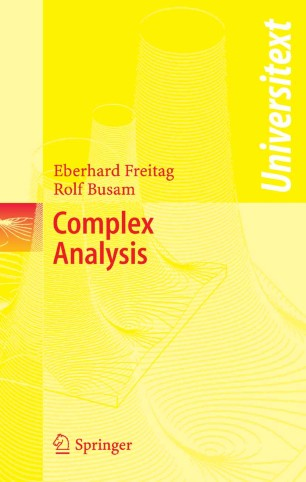# Complex Analysis

• Eberhard Freitag
• Rolf BusamTextbook

Part of the Universitext book series (UTX)

1. Front Matter
Pages I-X
2. Pages 1-7
3. Pages 71-104
4. Pages 195-255
5. Pages 257-325
6. Pages 327-390
7. Pages 391-458
8. Pages 459-521
9. Back Matter
Pages 523-552

### Introduction

The guiding principle of this presentation of ``Classical Complex Analysis'' is to proceed as quickly as possible to the central results while using a small number of notions and concepts from other fields. Thus the prerequisites for understanding this book are minimal; only elementary facts of calculus and algebra are required.

The first four chapters cover the essential core of complex analysis:

-  differentiation in  C  (including elementary facts about conformal mappings)
-  integration in C   (including complex line integrals, Cauchy's Integral Theorem, and the Integral Formulas)
-  sequences and series of analytic functions,   (isolated) singularities, Laurent series, calculus of residues
-  construction of analytic functions:  the gamma function, Weierstrass' Factorization Theorem, Mittag-Leffler Partial Fraction Decomposition, and -as a particular highlight- the Riemann Mapping Theorem, which characterizes the simply connected domains in C.

Further topics included are:

- the theory of elliptic functions based on the model of K. Weierstrass (with an excursions to older approaches due to N.H. Abel and C.G.J. Jacobi using theta series)
-  an introduction to the theory of elliptic modular functions and elliptic modular forms
-  the use of complex analysis to obtain number theoretical results
-  a proof of the Prime Number Theorem with a weak form of the error term.

The book is especially suited for graduated students in mathematics and advanced undergraduated students in mathematics and other sciences.

Motivating introductions, more than four hundred exercises of all levels of difficulty with hints or solutions, historical annotations, and over 120 figures make the overall presentation very attractive. The structure of the text, including abstracts beginning each chapter and highlighting of the main results, makes this book very appropriate for self-guided study and an indispensable aid in preparing for tests.

This English edition is based on the fourth forthcoming German edition.

### Keywords

Complex analysis Elliptic Functions Elliptic Modular Forms Modular form Residue theorem calculus minimum

#### Authors and affiliations

• Eberhard Freitag
• 1
• Rolf Busam
• 1
1. 1.Faculty of Mathematics, Institute of MathematicsUniversity of HeidelbergHeidelbergGermany